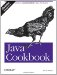# Recipe 5.18 Using Complex Numbers

#### Problem

You need to manipulate complex numbers, as is common in mathematical, scientific, or engineering applications.

#### Solution

Java does not provide any explicit support for dealing with complex numbers. You could keep track of the real and imaginary parts and do the computations yourself, but that is not a very well-structured solution.

A better solution, of course, is to use a class that implements complex numbers. I provide just such a class. First, an example of using it:

`// ComplexDemo.java  Complex c = new Complex(3,  5);  Complex d = new Complex(2, -2);  System.out.println(c + ".getReal( ) = " + c.getReal( ));  System.out.println(c + " + " + d + " = " + c.add(d));  System.out.println(c + " + " + d + " = " + Complex.add(c, d));  System.out.println(c + " * " + d + " = " + c.multiply(d));`

Example 5-4 is the complete source for the Complex class and shouldn't require much explanation. To keep the API general, I provide for each of add, subtract, and multiply both a static method that works on two complex objects and a nonstatic method that applies the operation to the given object and one other object.

##### Example 5-4. Complex.java
`/** A class to represent Complex Numbers. A Complex object is   * immutable once created; the add, subtract and multiply routines   * return newly created Complex objects containing the results.   *   */  public class Complex {      /** The real part */      private double r;      /** The imaginary part */      private double i;      /** Construct a Complex */      Complex(double rr, double ii) {          r = rr;          i = ii;      }      /** Display the current Complex as a String, for use in       * println( ) and elsewhere.       */      public String toString( ) {          StringBuffer sb = new StringBuffer( ).append(r);          if (i>0)              sb.append('+');    // else append(i) appends - sign          return sb.append(i).append('i').toString( );      }      /** Return just the Real part */      public double getReal( ) {          return r;      }      /** Return just the Real part */      public double getImaginary( ) {          return i;      }      /** Return the magnitude of a complex number */      public double magnitude( ) {          return Math.sqrt(r*r + i*i);      }        /** Add another Complex to this one */      public Complex add(Complex other) {          return add(this, other);      }      /** Add two Complexes */      public static Complex add(Complex c1, Complex c2) {          return new Complex(c1.r+c2.r, c1.i+c2.i);      }        /** Subtract another Complex from this one */      public Complex subtract(Complex other) {          return subtract(this, other);      }      /** Subtract two Complexes */      public static Complex subtract(Complex c1, Complex c2) {          return new Complex(c1.r-c2.r, c1.i-c2.i);      }        /** Multiply this Complex times another one */      public Complex multiply(Complex other) {          return multiply(this, other);      }      /** Multiply two Complexes */      public static Complex multiply(Complex c1, Complex c2) {          return new Complex(c1.r*c2.r - c1.i*c2.i, c1.r*c2.i + c1.i*c2.r);      }      /** Divide c1 by  c2.       * @author Gisbert Selke.      */     public static Complex divide(Complex c1, Complex c2) {         return new Complex(         (c1.r*c2.r+c1.i*c2.i)/(c2.r*c2.r+c2.i*c2.i),         (c1.i*c2.r-c1.r*c2.i)/(c2.r*c2.r+c2.i*c2.i));     } }`Java Cookbook, Second Edition
ISBN: 0596007019
EAN: 2147483647
Year: 2003
Pages: 409
Authors: Ian F Darwin

Similar book on Amazon